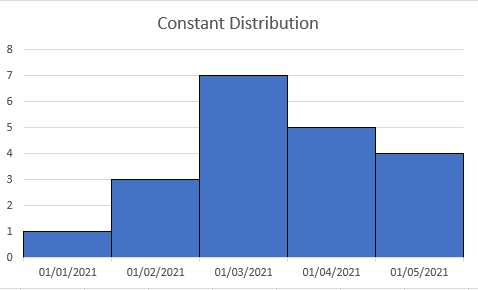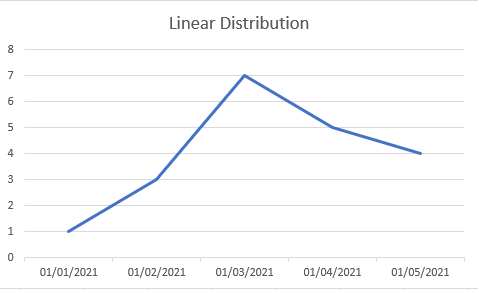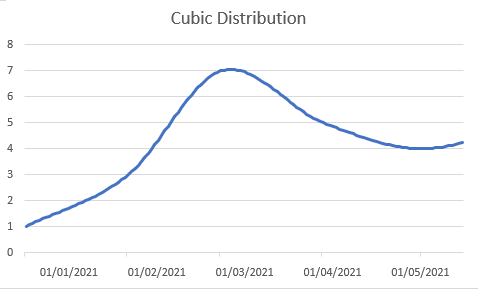# TimeSeries Scaling

This guide explains how to rescale TimeSeries to a different calendar.

## Introduction​

Rescaling TimeSeries is the process of changing the changing the calendar of the TimeSeries and fitting the data using that new calendar.

Rescaling from a high frequency calendar to a low frequency calendar, e.g. Daily to Monthly is called `aggregation`

Rescaling from a low frequency calendar to a high frequency calendar, e.g. Monthly to Daily is called `distribution`

## Aggregation​

When you rescale data from a high frequency calendar to a low frequency calendar, we need to reduce or aggregate the data. The way the data is aggregated is determined by the `observed` setting.

### Observed setting​

#### beginning​

An observation made at the beginning of the period

#### end​

An observation made at the end of the period.

This is typically used for end of day settlement prices where the settlement value is either the last traded price or a calculation using the last tradded prices.

#### averaged​

An average of the values observed throughout the period

#### summed​

A sum of the values observed throughout the period

This is typically used for volumes, e.g. the number of traded items in a day, so aggregating to a lower frequency will sum up these values to give you a total volume for the period.

#### high​

The highest value observed throuhout the period

#### low​

The lowest value observed throughout the period

#### delta​

The change of value from the start to the end of the period.

This is typically used for metered data where the values are meter readings which always increase in value. Scaling using delta allows you to see the amount used per period.

#### none​

A point-in-time observation

### Implicit observed​

When you scale a TimeSeries without specifying the observed option, OpenDataDSL first checks the global observed setting which is set using:

``set observed value``

If this hasn't been set, it uses the observed attribute of the TimeSeries which defaults to `end`.

## Distribution​

When you rescale data from a low frequency calendar to a high frequency calendar, we need to distribute the observed value across a range of values. The way the data is distributed is determined by the `distribution` setting.

### Distribution setting​

#### constant​

The value is constant across the whole period.

This can be seen like a bar chart where the tops of the bars are flat and the value jumps at each source period start.#### linear​

The values are interpolated using a linear spline between the source values.

This can be seen as a simple line chart where the values are points on the line#### cubic​

The values are interpolated using a cubic spline between the source values

This can be seen as a smoothed line chart.#### none​

A single value is used at the source index, the rest of the values are filled in with NaN or Missing

### Implicit distribution​

When you scale a TimeSeries without specifying the distribution method, OpenDataDSL first checks the global distribution setting which is set using:

``set distribution value``

If this hasn't been set then `constant` distribution is used.

### Distribution value​

The value used to calculate the distributed values is the observed value in the source TimeSeries except for the following cases:

#### Summed observed​

If the TimeSeries is observed as `summed` then the distributed values are divided by the number of observations so that the sum of those observations matches the original observed value.

## Scaling​

To rescale a TimeSeries, you using the `scale` function which has 3 signatures:

``ts = scale(input, calendar)ts = scale(input, calendar, observed)ts = scale(input, calendar, observed, distribution)``

### Examples​

#### Monthly to Daily TimeSeries​

``// Create a monthly TimeSeriesmts = TimeSeries("2021-01-01", MonthlyCalendar(), [1,3,7,5,4,5])// Scale to daily using a cubic splinects = scale(mts, DailyCalendar())print cts.values``

#### Monthly to Daily TimeSeries - cubic spline​

``// Create a monthly TimeSeriesmts = TimeSeries("2021-01-01", MonthlyCalendar(), [1,3,7,5,4,5])// Scale to daily using a cubic splinects = scale(mts, DailyCalendar(), "beginning", "cubic")print cts.values``